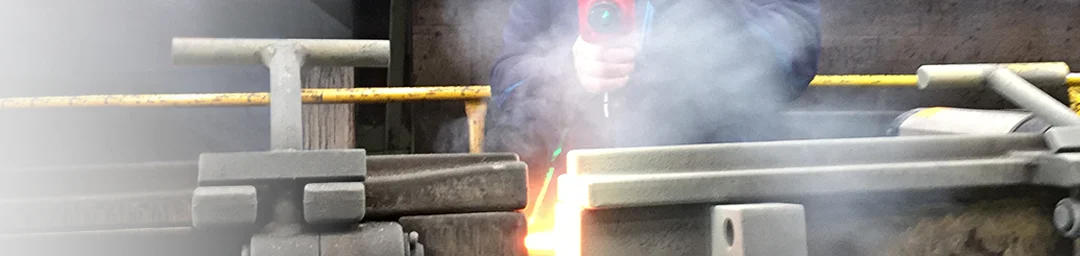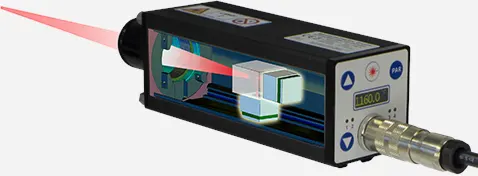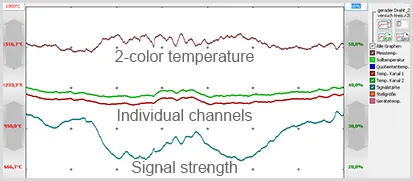# 2-color pyrometers

2-color pyrometers measure the infrared radiation at two closely spaced wavelengths at the same time and determine the temperature by forming a quotient.

Such devices are often used for measurements through dirty windows, steam or dust in the pyrometer's field of view. Even if the measuring object is smaller than the pyrometer measuring field or the emissivity of the material is unknown or an exact determination is not possible.Our 2-color pyrometers are characterized by:

• 2 separate measuring detectors for the two spectral ranges for reliable recording of measured values even with low signal strengths.
• Digital microcontroller signal processing for 100% reproducibility of the displayed measured values.
• IR signal monitoring for warning if the optics or windows are too dirty.

The ratio technique also allows the determination of very special applications such as pouring stream measurement or the flame measurement. Intelligent algorithms are also integrated here, which output a reasonable measurement result from the complex interaction of the individual signals, the signal strengths and the different emissivity of the measurement wavelengths.Always included, SensorTools software:
to display and evaluate the

• 2-color temperature
• Temperatures of the individual measurement spectral ranges
• Signal strength

2-color pyrometers are available in the following series:

FAQ

### What role does the distance between the two wavelengths play?

An independent measurement is carried out in each wavelength range, which normally requires the emissivity of the measuring material. However, since this is cut out again when the relationship is formed, it is on the other hand not necessary to know it.

However, a prerequisite for an error-free measurement result is an always constant ratio, even if the measurement temperature changes. With many materials, however, the emissivity changes depending on the temperature or even the measurement angle. The further the two measurement wavelengths are apart, the more the ratio changes in such materials when the temperature changes. The measurement deviations are then of different sizes, depending on which temperature is currently being measured. Measuring devices with spectral ranges that are further apart are only suitable for measurements on so-called "gray radiators", which are usually not available.

The measuring wavelengths of Sensortherm's 2-color pyrometers are close to each other in order to keep this error as low as possible. The individual measurement results of the two spectral ranges are therefore more parallel, which is necessary in order to always correctly calculate the quotient at all measurement temperatures.

### When do I have to adjust the emissivity slope?

Measurement objects that emit the same at the two wavelengths of the 2-color pyrometer are often described as "gray body" (e.g. well-oxidized iron or steel surfaces). They can be measured precisely without correction with the setting 1.00 for the emissivity slope.
Measuring objects whose emissivity is different at the two wavelengths of the quotient pyrometer (e.g. bare, non-oxidized metal surfaces) require a correction setting for an accurate temperature measurement, the so-called emissivity ratio Ɛ2 / Ɛ1. The value can be greater or less than 1, depending on whether the emissivity of channel 1 or 2 is higher (gain 1.000–0.800; attenuation 1.000–1.200).

Regardless of whether the measurement is made with an emissivity slope of 1 or above or below, the ratio must remain constant over the required temperature range. In the SensorTools software, the 2-color temperature and that of the two individual channels can be displayed at the same time. If the curves of the two individual channels run uniformly parallel in the required measuring range, the ratio is constant and a 2-color measurement is possible. If the temperature values of the curves are the same (with the same set emissivity), the emissivity ratio is 1. If they run in parallel, the correct value for the emissivity slope must be found, e.g. by a comparison measurement with a thermocouple and subsequent ratio adjustment until the temperatures match.